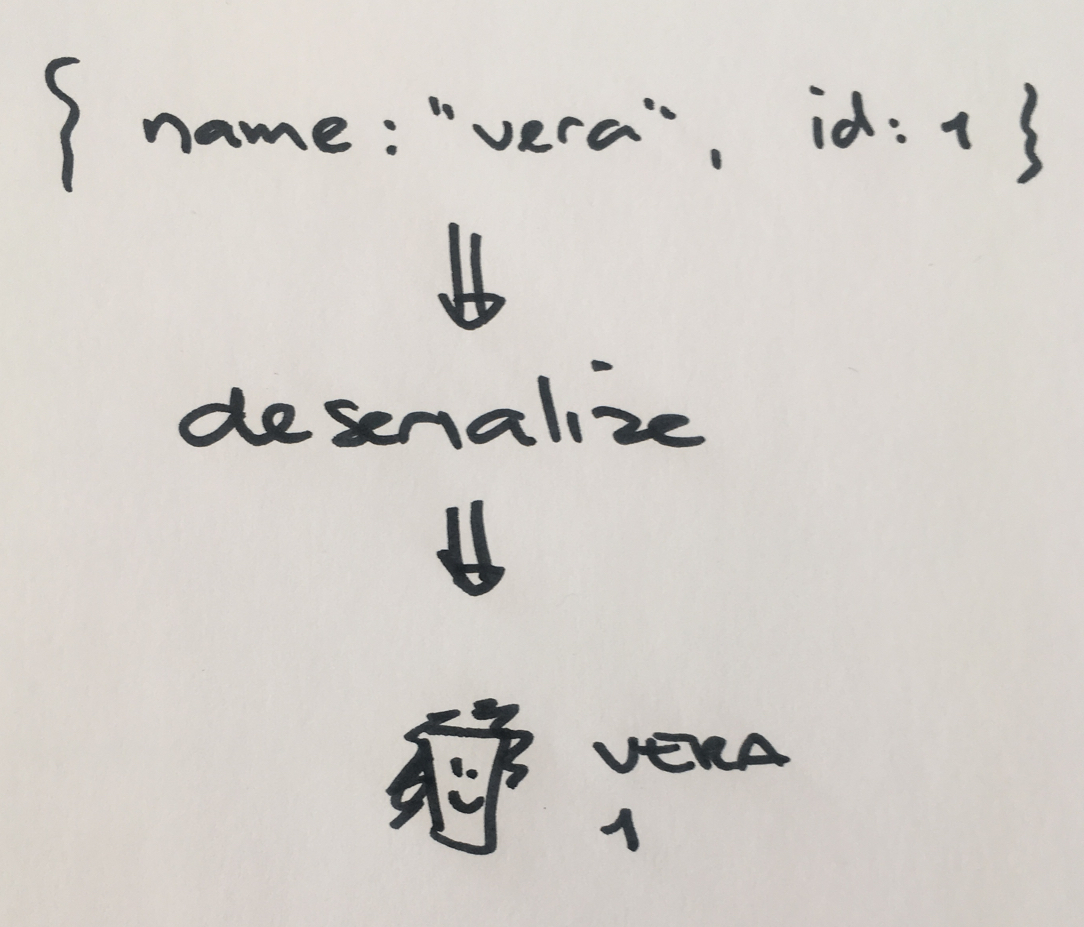# Create object equality in Javascript

Are you looking for a way to check if two objects represent the same data in javascript? Perhaps you are here because you were sure two variables had the same value, only to discover they were just references to two different objects. How can you deal with comparing objects in Javascript? After reading this, you understand why objects that look the same, might be considered not the same and how to solve this problem.

When comparing variables in most programming languages, there is a difference between comparing values and comparing objects. Here is an example to compare two strings in Javascript:

``````let v1 = "vera"
let v2 = "vera"
let c1 = "chuck"
console.log(v1 === v2);
console.log(v1 === c1);
``````

result:

``````true
false
``````

As expected, if you compare strings `"vera"` to `"vera"`, the result is true. Now let’s create a class that holds the id and name of the employee:

``````class Employee {
constructor(id, name) {
this.id = id;
this.name = name;
}
}
``````

This allows you to create employees like Vera:• Create the same employees as before and check for equality:
``````let v1 = new Employee(1, "Vera");
let v2 = new Employee(1, "Vera");
let c1 = new Employee(2, "Chuck");
console.log(v1 === v2);
console.log(v1 === c1);
``````

result:

``````false
false
``````

Perhaps you already expected both compare results to be false. But why is that? And when is this going to be important?

• Create a list of employees
• Make a variable that points to the first item
``````let employees = [
new Employee(1, "Vera"),
new Employee(2, "Chuck"),
new Employee(3, "Dave"),
]
let vera = employees;
``````If you compare the first item of the list to the variable `vera`, the result is true because both point to the same object:

``````console.log(employees === vera);
``````

result:

``````true
``````## Equality after deserializing

A common scenario is serializing and deserializing objects. For many different reasons you might construct an object from json or a html data attribute.The object created from deserializing, is not the same object as the original object in your lists.Using `==` or `===` to compare the two objects is not going to produce the result you want. You need to check for equality in a different way.

## Solution: Create equals function

Start by adding a hash function that returns a unique identifier for an object. In our case, an employee has an `id` that we consider unique. The hash function return the id.

## Step 1: hash function

``````class Employee {
constructor(id, name) {
this.id = id;
this.name = name;

this.hash = () => this.id;
}
}
``````
``````let vera = new Employee(1, "Vera")
console.log(vera.hash());
``````

result:

``````1
``````

### Step 2: equals function

``````class Employee {
constructor(id, name) {
this.id = id;
this.name = name;

this.hash = () => this.id;
this.equals = (other) => {
if (other === null && this !== null) {
return false;
}
return this.hash() === other.hash();
}
}
}
``````

Now variables that point to different objects –but represent the same data– can be checked for equality like this:

``````let vera1 = new Employee(1, "Vera")
let vera2 = new Employee(1, "Vera")
let chuck1 = new Employee(2, "Chuck")
console.log(vera1.equals(vera2));
console.log(vera1.equals(chuck1));
``````

result:

``````true
false
``````

If at a later moment, your class needs to be unique in a different way, you can change the hash function without touching the equals function.

Grüsse aus Berlin!
This is a cookie free and popup free website.Join me in making the web enjoyable again by removing the need for annoying cookie warnings and silly newsletter overlays!